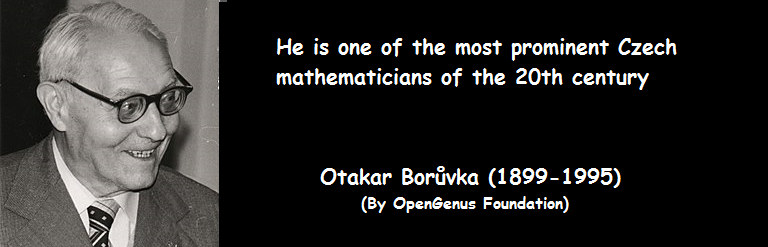×

Search anything:

# Boruvka Minimum Spanning Tree

#### Graph Algorithms minimum spanning tree boruvka minimum spanning treeGet this book -> Problems on Array: For Interviews and Competitive Programming

Reading time: 15 minutes | Coding time: 10 minutes

Borůvka's algorithm is an algorithm for finding a minimum spanning tree in a graph for which all edge weights are distinct, or a minimum spanning forest in the case of a graph that is not connected.In fact, this is the first algorithm developed to find minimum spanning tree and its origin dates back to 1926.

The credit of Boruvka's algorithm goes to Otakar Borůvka, Choquet, Florek, Łukasiewicz, Perkal, Steinhaus and Zubrzycki and Georges Sollin.

Steps involved are:

1. Input graph is a connected, weighted and directed graph.
2. Initialize all vertices as individual components (or sets).
3. Initialize Minimum Spanning Tree (MST) as empty.
4. While there are more than one components, do following
for each component.
a) Find the closest weight edge that connects this component to any other component.
5. Return Minimum Spanning Tree (MST).

### Complexity

• Worst case time complexity: `Θ(E log V)`
• Average case time complexity: `Θ(E log V)`
• Best case time complexity: `Θ(E log V)`
• Space complexity: `Θ(E + V)`

• C++

### C

``````
#include <iostream>
#include <vector>
const int MAXN = 10000;
const int inf = 0x3fffffff;
int nodeCount, edgesCount;
std::vector<std::pair<int, int> > graf[MAXN];
std::cin >> nodeCount >> edgesCount;
for (int i = 0; i < edgesCount; i++) {
int x, y, w;
std::cin >> x >> y >> w;
graf[x].push_back({y, w});
graf[y].push_back({x, w});
}
}
int parent[MAXN], rank[MAXN];
// Initialize the forest of trees
void initDisjoint() {
for (int i = 0; i < nodeCount; i++) {
parent[i] = i;
rank[i] = 0;
}
}
// Get set representative
int getSR(int x) {
if (x == parent[x])
return x;
return parent[x] = getSR(parent[x]);
}
int areUnited(int x, int y) {
return getSR(x) == getSR(y);
}
void unite(int x, int y) {
x = getSR(x);
y = getSR(y);
if (rank[x] > rank[y])
parent[y] = x;
else {
parent[x] = y;
if (rank[x] == rank[y])
rank[y]++;
}
}
std::pair<int, int> cheapEdge[MAXN];
int solve() {
int numberOfTrees = nodeCount;
int weightSum = 0;
initDisjoint();
while (numberOfTrees > 1) {
// initializing cheapest edge to "none"
for (int i = 0; i < nodeCount; i++)
cheapEdge[i].first = -1;
for (int i = 0; i < nodeCount; i++) {
for (size_t j = 0; j < graf[i].size(); j++) {
std::pair<int, int> edge = graf[i][j];
int xsr = getSR(i);
int ysr = getSR(edge.first);
int w = edge.second;
if (xsr == ysr) continue;
if (cheapEdge[xsr].first == -1 || w < graf[cheapEdge[xsr].first][cheapEdge[xsr].second].second)
cheapEdge[xsr] = {i, j};
if (cheapEdge[ysr].first == -1 || w < graf[cheapEdge[ysr].first][cheapEdge[ysr].second].second)
cheapEdge[ysr] = {i, j};
}
}
for (int i = 0; i < nodeCount; i++) {
int xsr = getSR(i);
if (cheapEdge[xsr].first == -1) continue;
std::pair<int, int> edge = graf[cheapEdge[xsr].first][cheapEdge[xsr].second];
int ysr = getSR(edge.first);
if (xsr == ysr) continue;
weightSum += edge.second;
unite(xsr, ysr);
numberOfTrees--;
}
}
return weightSum;
}
int main() {
int weight = solve();
std::cout << "The weight of the minimum spanning tree is " << weight;
return 0;
}
``````

### Applications

Applications of Borůvka's minimum spanning tree algorithm are:

• Used to find the Minimum Spanning Tree using a greedy approach
• Boruvka’s algorithm is used as a step in a faster randomized algorithm that works in linear time O(E)
• See Applications of Minimum Spanning Tree